IMO Shortlist 2010 problem C2

Kvaliteta:
Avg: 0,0
Težina:
Avg: 6,0
On some planet, there are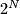$2^N$ countries$(N \geq 4).$ Each country has a flag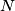$N$ units wide and one unit high composed of$N$ fields of size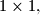$1 \times 1,$ each field being either yellow or blue. No two countries have the same flag. We say that a set of$N$ flags is diverse if these flags can be arranged into an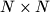$N \times N$ square so that all$N$ fields on its main diagonal will have the same color. Determine the smallest positive integer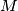$M$ such that among any$M$ distinct flags, there exist$N$ flags forming a diverse set.

Proposed by Tonći Kokan, Croatia
Izvor: Međunarodna matematička olimpijada, shortlist 2010